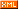# 挽星

:: :: :: :: :: :::: ::190 随笔 :: 204 文章 :: 1 评论 :: 0 引用

importjava.util.Date;

importjava.util.Random;

publicclass LotteryTool {

private double factor;

private double probability;

private Random rand;

private LotteryTool(double probability, long expireTime, int reduce){

this.factor = (double) System.currentTimeMillis() / expireTime;

this.probability = probability * Math.pow(factor, reduce);

this.rand = new Random(System.currentTimeMillis());

}

public static LotteryTool getInstance(double probability, longexpireTime,

int reduce) {

return new LotteryTool(probability, expireTime, reduce);

}

public boolean isLucky(long expected) {

long token = generateLong();

expected = expected % (int) (1 / probability);

if (expected == token) {

return true;

}

return false;

}Alias Method 复杂度：预处理O(NlogN)，随机数生成O(1)，空间复杂度O(2N)

posted on 2013-08-10 10:59  挽星  阅读(89031)  评论(0编辑  收藏